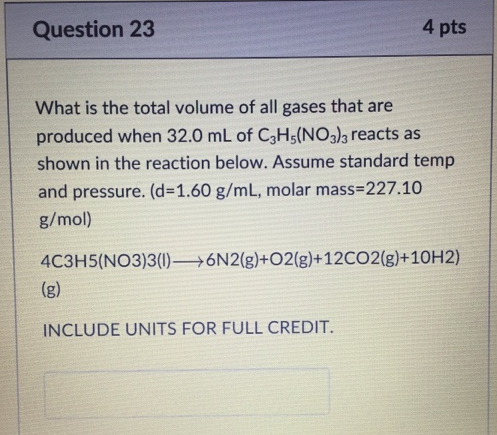# Problem: What is the total volume of all gases that are produced when 32.0 mL of C3H5(NO3)3 reacts as shown in the reaction below. Assume standard temp and pressure. (d = 1.60 g/mL, molar mass 227.10 g/mol) 4C3H5(NO3)3 (I) → 6N2(g) + O2(g) + 12CO2(g) + 10H2 (g).

###### FREE Expert Solution
79% (160 ratings)###### Problem Details

What is the total volume of all gases that are produced when 32.0 mL of C3H5(NO3)3 reacts as shown in the reaction below. Assume standard temp and pressure. (d = 1.60 g/mL, molar mass 227.10 g/mol) 4C3H5(NO3)3 (I) → 6N2(g) + O2(g) + 12CO2(g) + 10H2 (g).